# First-Order Reactions: Definition & Mathematical Representation

Lesson Transcript
Instructor: Stephanie Bryan

Stephanie has a master's degree in Physical Chemistry and teaches college level chemistry and physics.

In this lesson, we'll talk about first-order reactions like radioactive decay. We'll use the mathematical descriptions of these reactions to discuss their behavior.

## Reaction Rates

How can scientists use uranium deposits to date the age of the earth? No one was alive back then - there weren't even bacteria! Well, it turns out the radioactive decay of Uranium-238 is very predictable and can be described mathematically, using first-order reactions.

The rate of reaction, or reaction rate, is the speed at which a reaction progresses. We define this mathematically by measuring the rate at which reactants disappear or products appear, where rate is defined as a derivative with respect to time. In this lesson, we're concentrating on first-order reactions. Because first-order reaction rates only depend on the concentration of one reactant, we can define the rate of these reactions as the rate of disappearance of this reactant.

Rate laws are equations that mathematically describe this rate. Knowing which variables determine the rate of reaction allows us to draw useful conclusions about that reaction. In this lesson, we'll look at first-order reactions, which depend only on the concentration of one reactant. We'll then use this rate law to derive an equation for the half-life of the reaction.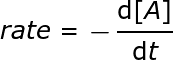In this expression, the brackets denote concentration, so A in brackets is the concentration of reactant A. The negative sign indicates that the concentration is going down over time, and t is time.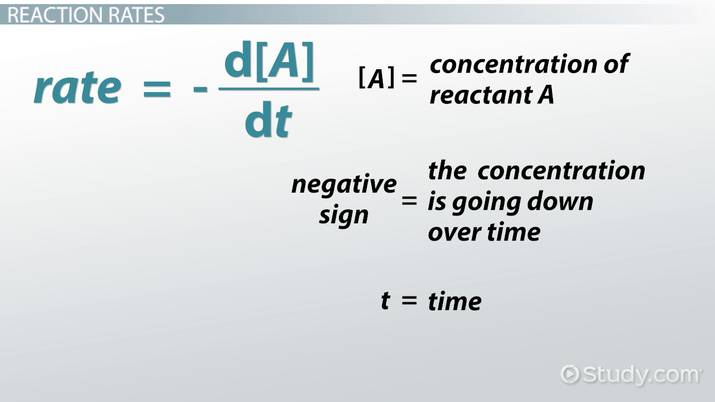An error occurred trying to load this video.

Try refreshing the page, or contact customer support.

Coming up next: Rate Constant and Rate Laws

### You're on a roll. Keep up the good work!

Replay
Your next lesson will play in 10 seconds
• 0:03 Reaction Rates
• 1:34 Differential Rate Law
• 2:21 Integrated Rate Law
• 3:15 Half-Life of First…
• 4:42 Sample Problem
• 6:21 Lesson Summary
Save Save

Want to watch this again later?

Timeline
Autoplay
Autoplay
Speed Speed

## Differential Rate Law

First-order reactions are only dependent on the concentration of one reactant raised to the power of one. In other words, in first-order reactions, the rate is proportional to the concentration of reactant A. If it were proportional to the concentration of two different reactants or to the concentration of reactant A-squared, it would be a second-order reaction. We will leave that discussion for another lesson.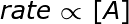We can turn a proportion into an equation by multiplying by a constant. This constant, k, is the reaction rate coefficient.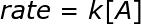Then, plugging the definition of rate from the previous section into our rate law gives us the differential rate law.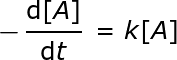## Integrated Rate Law

Starting with the differential rate law,we want to move all the concentrations to one side of the equation and move the constant and time to the other side of the equation. It can now be read as: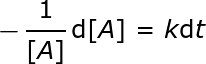Then we can integrate each side of the equation: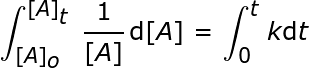Once we do that, it will give us the integral representation of the rate law: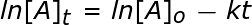With a bit of algebra, this can be represented in another way as well: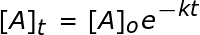## Half-Life of First-Order Reactions

The half-life of a reaction is the time that it takes to reduce the concentration of a reactant by half. In other words, at this point, the concentration of the reactant is half its initial concentration: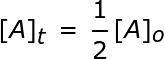If we plug this into our integrated rate law we would get the following equation for the half-life: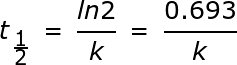To unlock this lesson you must be a Study.com Member.

### Register to view this lesson

Are you a student or a teacher?